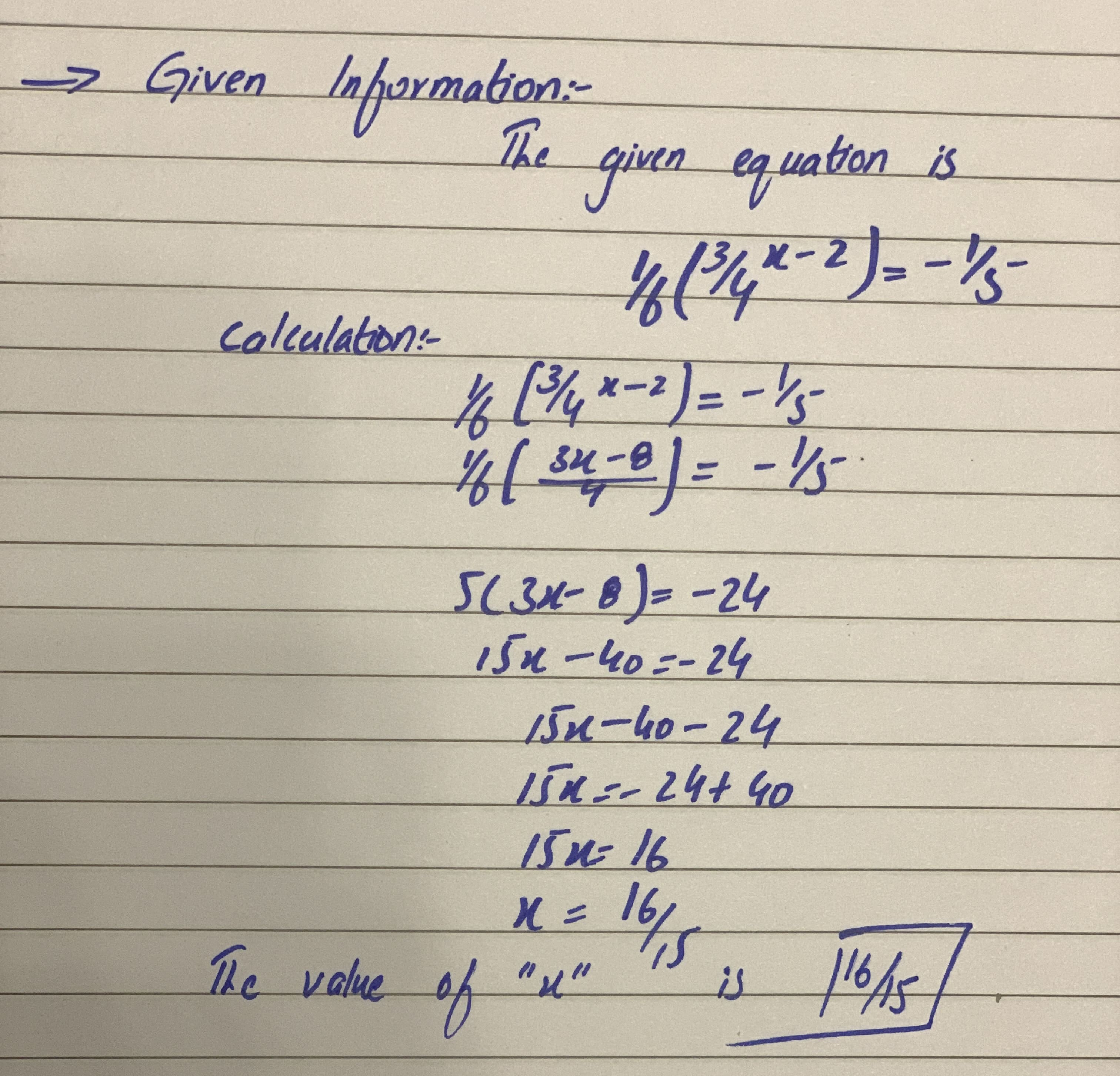# Solve the equations for x and simplify the answer if possible. Indicate which solving technique is used, Linear, Factor, SRP ZPR, QF.1/6(3/4x-2)=(-1)/5aflacatn 2021-01-17 Answered

Solve the equations for x and simplify the answer if possible. Indicate which solving technique is used, Linear, Factor, SRP ZPR, QF.
$\frac{1}{6}\left(\frac{3}{4x}-2\right)=\frac{-1}{5}$

You can still ask an expert for help

• Questions are typically answered in as fast as 30 minutes

Solve your problem for the price of one coffee

• Math expert for every subject
• Pay only if we can solve ityagombyeR

..............